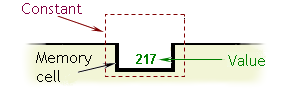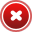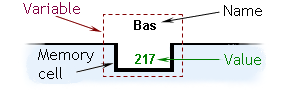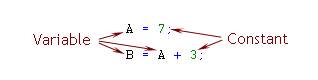English

MQL4 BookBasics of MQL4Constants and Variables

## Constants and variables

These two terms, constant and variable, are considered in one section since these terms are very close in themselves.

## The notion of constant

Part of a program, a constant is an object that has a value.

A constant in a program is similar to a constant used in mathematical equations. It is an invariable value. To describe the nature of a constant used in an algorithmic language in greater detail, let us refer to well-known physical and mathematical constants.

The human race has discovered natural, universal constants, the values of which do not depend on us in any way. For example, in physics, free fall acceleration is always equal to 9.8 m/s/s; in mathematics, Pi = 3.14. Constants of the kind are different than constants in an algorithmic language.

Constant is also a term used in mathematical equations. For example, in the equation of Y = 3 * X + 7, numbers 3 and 7 are constants. The values of such constants are fully dependent on the will of the person that has made the equation. This is the closest analogy of constants used in MQL4 programs.

A constant (as a value) is placed by a programmer in the code at the stage of its creation. The constant is characterized only by its value, so the terms of 'constant' and 'the value of a constant' are full synonyms.

### Exemplary constants:

`37, 3.14, true, "Kazan"`Figure 4 A Constant in the memory of a PC.

## The properties of constants

The property of a constant is its power to retain (during the time of the program operation) the value set by the programmer and to set this value to the program when the program requests it (Figure 5). For each constant in the program, the computer allocates a part of its memory of the necessary size. Neither the programmer nor the computer can change the value of a constant during execution of the program (Figure 6).The value of the constant remains the same.Figure 5 The state of the memory cell of a constant when setting the value to the program.The value of a constant cannot be changed during the program operation.Figure 6 It is impossible to change the value of a constant during the program operation.

## The notion of variable

A variable is a program part that has a value and a name.

The term of variable in MQL4 is similar to that accepted in mathematics. The difference between them consists only in that the value of a variable in mathematics is always implied, whereas the value of variable in an executing program is stored in a special memory cell in the computer.

The variable is put into the code text by its author at the stage of coding as a variable name. The name (or, identifier) of a variable can consist of letters, digits, or underscore. However, it must start with a letter. MQL4 is case-sensitive, that is "S" and "s" are not the same.

Here are some examples of variable names: Alpha, alFa, beta, NuMbEr, Num, A_37, A37, qwerty_123.

The following variable identifiers represent the names of different variables: a_22 and А_22; Massa and MASSA.

Here are some examples of variable values: 37, 3.14, true, "Kazan".

## The properties of variable

The property of a variable is its capability to get a certain value from the program, retain it during the period of operation of the program and set this value to the program when requested by the program. For each variable in the program, the computer allocates a part of its memory, a part with the necessary size. Let us refer to Figure 7 to study the construction of a variable.Figure 7 A Variable in the memory of a computer.

There is a value of a variable in the memory cell of the computer. This value can be requested for processing and changed by the program. The name of a variable is never changed. When writing a code, the programmer can set any name for the variable. However, as soon as the ready program is launched, neither programmer nor the computer, nor the program, has any way to change the name of the variable.

If, in the process of execution, a program meets the name of a variable, the program refers to this variable in order to get its value for processing. When a program refers to a variable, the variable sets a copy of its value to the program. At that point, the value of the variable remains the same, whereas the program gets the copy of the value contained in the memory cell allocated for this variable (Figure 8).When the value of a variable is set to a program, this value remains unchanged. Also, the name of a variable will never be changed.Figure 8 The state of the memory cell of a variable when setting the value to the program.

After an executing program has referred to a variable, the variable is not related to the program for a certain period of time until the program refers to it again. During this period, the program may refer to other variables or make necessary calculations. Between program references to the variable, the variable retains its value; that is, it keeps it unchanged.

According to the algorithm of the program, it can become necessary to change the value of a variable. In this case, the program sets to the variable its new value. The variable gets this value from the program. All necessary modifications are made in the memory cell itself. The result is that the preceding value of the variable is deleted, whereas a new value of the variable set by the program takes its place, as shown in Figure 9.The value of a variable can be changed by the program. But, the name of the variable is always unchanged.Figure 9 The state of the memory cell of a variable when getting the value from the program.

## Exemplary constants and variables in a program

In a program, constants and variables can be found in operators. In the code below, A and B are variables, and 7 and 3 are constants.

```A = 7; // Line 1
B = A + 3; // Line 2```

Let us study how a program works with constants and variables. Executing these lines, the program will make the following steps:

Line 1:

1.Constant 7 sets its value to the program.

2.Variable A gets value 7 from the program.

Line 2:

1.The program has found an expression to the right of the equality sign, and the program is trying to calculate it.

2.Constant 3 sets its value to the program.

3.The program refers to variable A by the name.

4.Variable A sets its value (7) to the program.

5.The program makes calculations (7 + 3).

6.Variable В gets value 10 from the program.

The value of a variable can be changed during the program operation. For example, there can be a line in the program that contains the following code.

`В = 33; // Line 3`

In this case, the following will be done at execution of the program:

1.Constant 33 sets its value to the program.

2.Variable B gets (new) value 33 from the program.

It is easy to notice that variable B gets value 10 at a certain stage of the program execution, and then it gets the value of 33. The name of variable B remains unchanged during all these events, whereas the value of the variable will change.

The following Figure 10 shows constants and variables in the program code.Figure 10 A constant and a variable in a program.Some Basic ConceptsData Types